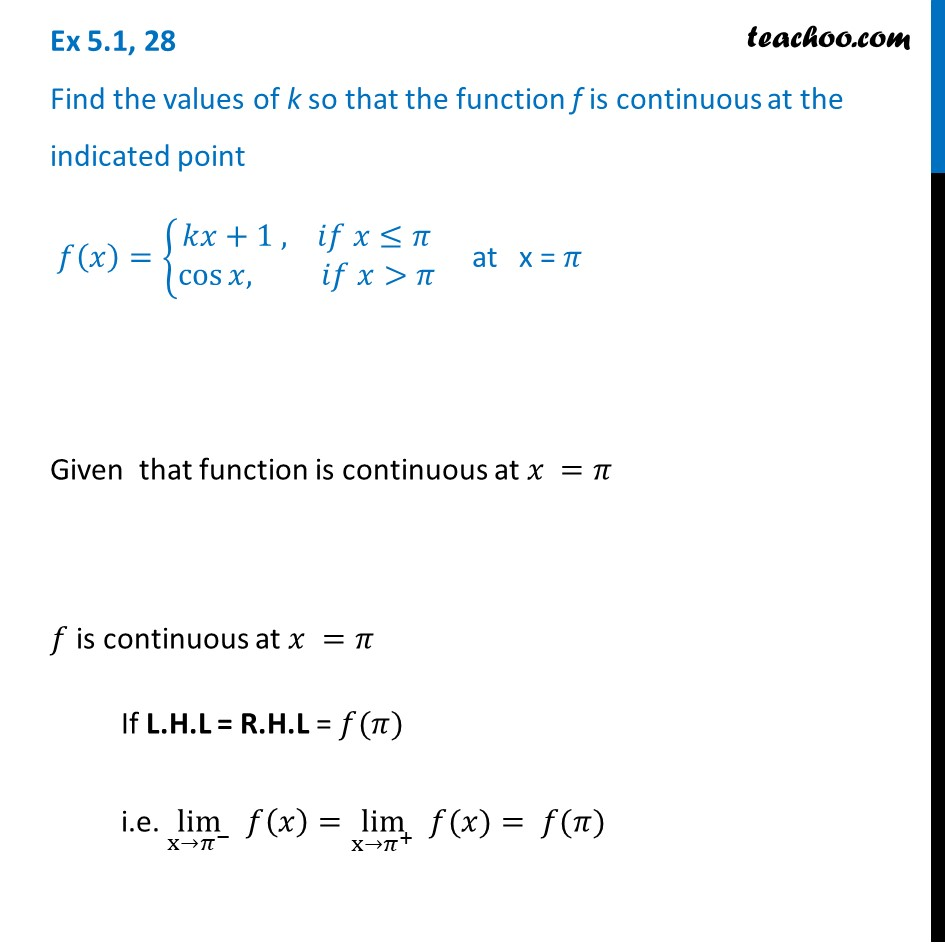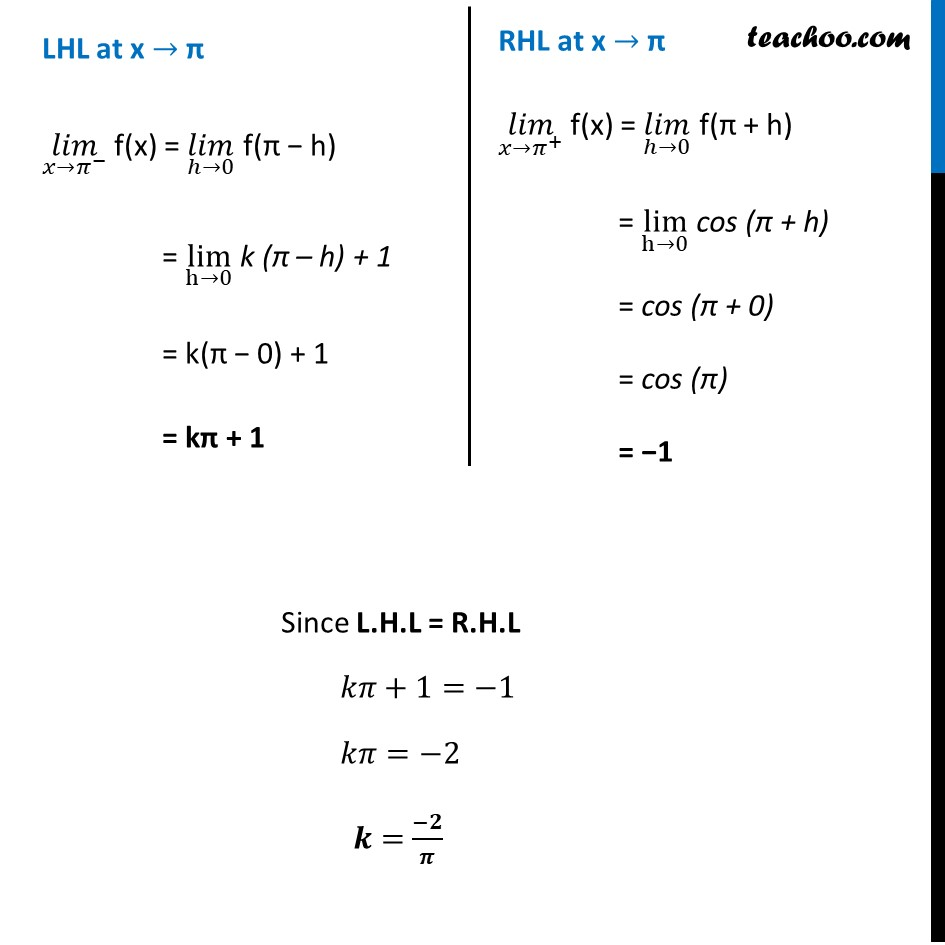Chapter 5 Class 12 Continuity and Differentiability

Class 12
Important Questions for exams Class 12Learn in your speed, with individual attention - Teachoo Maths 1-on-1 Class

### Transcript

Ex 5.1, 28 Find the values of k so that the function f is continuous at the indicated point 𝑓(𝑥)={█(𝑘𝑥+1 , 𝑖𝑓 𝑥≤𝜋@cos⁡〖𝑥, 〗 𝑖𝑓 𝑥>𝜋)┤ at x = 𝜋 Given that function is continuous at 𝑥 =𝜋 𝑓 is continuous at 𝑥 =𝜋 If L.H.L = R.H.L = 𝑓(𝜋) i.e. lim┬(x→𝜋^− ) 𝑓(𝑥)=lim┬(x→𝜋^+ ) " " 𝑓(𝑥)= 𝑓(𝜋) LHL at x → π (𝑙𝑖𝑚)┬(𝑥→𝜋^− ) f(x) = (𝑙𝑖𝑚)┬(ℎ→0) f(π − h) = lim┬(h→0) k (π – h) + 1 = k(π − 0) + 1 = kπ + 1 RHL at x → π (𝑙𝑖𝑚)┬(𝑥→𝜋^+ ) f(x) = (𝑙𝑖𝑚)┬(ℎ→0) f(π + h) = lim┬(h→0) cos (π + h) = cos (π + 0) = cos (π) = −1 Since L.H.L = R.H.L 𝑘𝜋+1=−1 𝑘𝜋=−2 𝒌= (−𝟐)/𝝅